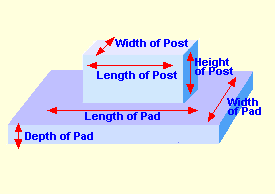Spike's Calculators

# Column and Rectangular Pad Concrete VolumeWith round columns, your pads are usually square, but when it calls for a square or rectangular column, the pad may have to be rectangular. With this calculator, you can add a depth, width, and length for the pad and the width, length, and height of the column using feet and inches for measurements.

Number of Columns and Pads:  #
Length of Column: in
Width of Column:  in.
Height of Column:  ft in

Cubic Feet: ft³
Cubic Yards: yd³
Cubic Meters:

#### Calculation

1. enter the number of columns and pads you need
2. length of the column in inches
3. the width of the column in inches
4. the height of the column in feet and inches
5. the pad length in feet and inches
6. the width of the pad in feet and inches
7. the depth of the pad in inches

#### Results

1. the amount of concrete needed in cubic feet
2. in cubic yards
3. in cubic metres

### Conversions

```one cubic inch (in³) = 0.000578703703703704 cubic feet (ft³)
one cubic inch (in³) = 0.0000214334705075445 cubic yards (yd³)
one cubic inch (in³) = 0.000016387064 cubic metres (m³)```
```To convert cubic feet to cubic yards, multiply cubic feet * 0.037037037037037
To convert cubic feet to cubic metres, multiply cubic feet * 0.028316846592
To convert cubic yards to cubic metres, multiply cubic yards * 0.764554857984```

### When Ordering Concrete

This concrete calculator will help you in estimating the amount of concrete needed for your project. The amount given as needed, does not include any waste. It is recommended, depending on the job you are doing, to add anywhere from an extra 4% to 10% to your concrete order to make sure you have enough concrete to finish the job. Ask when ordering the concrete. They will be able to give you a good idea of what is needed.